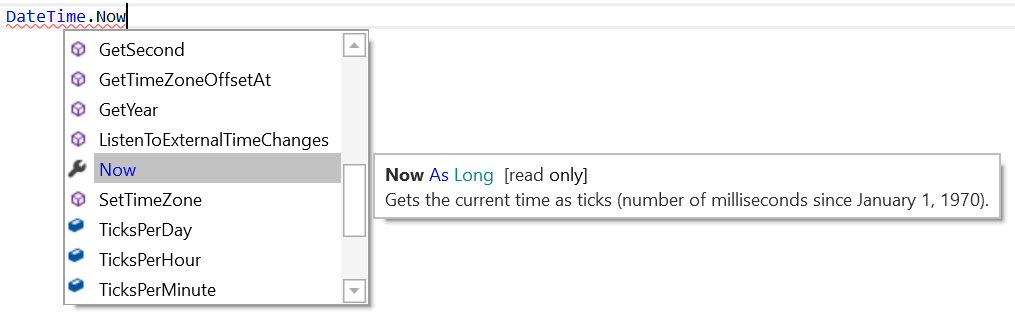# Android QuestionWrong Long Data Type

#### RobertoS

##### Member
Longtime User
I have created a function to count my seconds since January 30, 1899.
This function returns a result that is over 3,000,000,000. I thought of using a long, but when effect multiplication has a negative result.

What kind of data should I use?
I also tried with float and double without success.

The code:
B4X:
``````Sub getTikker() As Long

Dim p As Period

Dim oggi As Long = DateTime.Now
Dim inizio As Long = DateUtils.SetDateAndTime(DateTime.GetYear(oggi), DateTime.GetMonth(oggi), DateTime.GetDayOfMonth(oggi), _
DateTime.GetHour(oggi),DateTime.GetMinute(oggi), DateTime.GetSecond(oggi))
Dim fine As Long = DateUtils.SetDateAndTime(1899, 12, 30, 23, 59 ,59)

p = DateUtils.PeriodBetweenInDays(fine, inizio)

Dim tmp As Long = p.Days * 86400 + p.Hours * 3600 + p.Minutes * 60 + p.Seconds
Log("long: " & tmp)

Dim tmp2 As Double = p.Days * 86400 + p.Hours * 3600 + p.Minutes * 60 + p.Seconds
Log("double: " & tmp2)

Log("simple long data type test")
Log("43872 * 86400 = " & (43872 * 86400)) ' simple long test

Return tmp

End Sub``````

Log Result
B4X:
``````Logger connesso a:  samsung SM-A405FN
--------- beginning of main
*** Service (starter) Create ***
** Service (starter) Start **
** Activity (main) Create, isFirst = true **
long: -504470928
double: -5.04470928E8
simple long data type test
43872 * 86400 = -504426496
** Activity (main) Resume **``````
Thanks

#### aeric

##### Expert
Longtime User
The reason you get negative value is DateTime.Now returns value since 1 Jan 1970.•DonManfred

#### stevel05

##### Expert
Longtime User
•Peter Simpson and RobertoS

Longtime User

#### stevel05

##### Expert
Longtime User
It returns the same Long value (already had the ide with bignumbers and tried)
Yes, I guess it would as it's cast to a Long.

I don't know if it would work dividing by 10000 or so and returning a double, then formatting the output maybe I'll try it in a bit.

Longtime User

#### JordiCP

##### Expert
Longtime User
If any of the factors involved in the calculations is an int, as it seems to happen with constants, the partial result is casted into an int. Try working with only longs
B4X:
``````    ' Partial results are casted into an int (32bit)'
Log("43872 * 86400 = " & (43872 * 86400)) ' Log --> 43872 * 86400 = -504426496

' All factors are long (64bit), so no way that partial results are casted to int
Dim n1 As Long = 43872
Dim n2 As Long = 86400
Log("43872 * 86400 = " & (n1 * n2)) ' Log --> 43872 * 86400 = 3790540800``````

#### RobertoS

##### Member
Longtime User
If any of the factors involved in the calculations is an int, as it seems to happen with constants, the partial result is casted into an int. Try working with only longs
B4X:
``````    ' Partial results are casted into an int (32bit)'
Log("43872 * 86400 = " & (43872 * 86400)) ' Log --> 43872 * 86400 = -504426496

' All factors are long (64bit), so no way that partial results are casted to int
Dim n1 As Long = 43872
Dim n2 As Long = 86400
Log("43872 * 86400 = " & (n1 * n2)) ' Log --> 43872 * 86400 = 3790540800``````

Thank's!!!